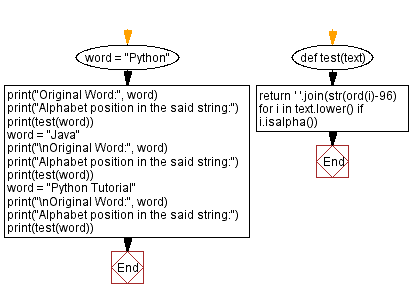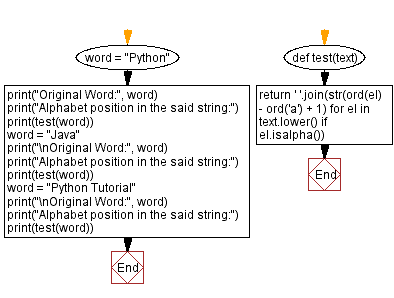﻿ Python - Alphabet position in a string# Python Exercises: Alphabet position in a string

## Python String: Exercise-111 with Solution

Write a Python program that takes a string and replaces all the characters with their respective numbers.

Sample Data:
("Python") -> "16 25 20 8 15 14"
("Java") -> "10 1 22 1"
(“Python Tutorial”) -> "16 25 20 8 15 14 20 21 20 15 18 9 1 12"

Sample Solution-1:

Python Code:

``````def test(text):
return ' '.join(str(ord(i)-96) for i in text.lower() if i.isalpha())
word = "Python"
print("Original Word:", word)
print("Alphabet position in the said string:")
print(test(word))
word = "Java"
print("\nOriginal Word:", word)
print("Alphabet position in the said string:")
print(test(word))
word = "Python Tutorial"
print("\nOriginal Word:", word)
print("Alphabet position in the said string:")
print(test(word))
```
```

Sample Output:

```Original Word: Python
Alphabet position in the said string:
16 25 20 8 15 14

Original Word: Java
Alphabet position in the said string:
10 1 22 1

Original Word: Python Tutorial
Alphabet position in the said string:
16 25 20 8 15 14 20 21 20 15 18 9 1 12
```

Flowchart:## Visualize Python code execution:

The following tool visualize what the computer is doing step-by-step as it executes the said program:

Sample Solution-2:

Python Code:

``````def test(text):
return ' '.join(str(ord(el) - ord('a') + 1) for el in text.lower() if el.isalpha())
word = "Python"
print("Original Word:", word)
print("Alphabet position in the said string:")
print(test(word))
word = "Java"
print("\nOriginal Word:", word)
print("Alphabet position in the said string:")
print(test(word))
word = "Python Tutorial"
print("\nOriginal Word:", word)
print("Alphabet position in the said string:")
print(test(word))
```
```

Sample Output:

```Original Word: Python
Alphabet position in the said string:
16 25 20 8 15 14

Original Word: Java
Alphabet position in the said string:
10 1 22 1

Original Word: Python Tutorial
Alphabet position in the said string:
16 25 20 8 15 14 20 21 20 15 18 9 1 12
```

Flowchart:## Visualize Python code execution:

The following tool visualize what the computer is doing step-by-step as it executes the said program:

Python Code Editor:

Have another way to solve this solution? Contribute your code (and comments) through Disqus.

Previous Python Exercise: Insert space before capital letters in word.
Next Python Exercise: Calculate the sum of two numbers given as strings.

What is the difficulty level of this exercise?

Test your Programming skills with w3resource's quiz.

﻿

## Python: Tips of the Day

Getting sorted iterable (can sort by "compare" function):

```>>> a = [1, 2, -3]
>>> sorted(a)
[-3, 1, 2]

>>> sorted(a,key=abs)
[1, 2, -3]
```